# Mass and Motion Mass and Force Mass is

• Slides: 6Mass and Motion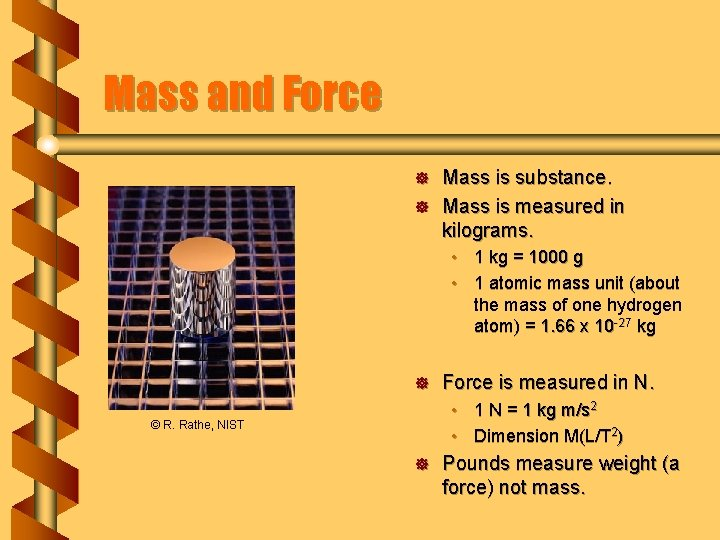Mass and Force ] ] Mass is substance. Mass is measured in kilograms. • 1 kg = 1000 g • 1 atomic mass unit (about the mass of one hydrogen atom) = 1. 66 x 10 -27 kg ] Force is measured in N. • 1 N = 1 kg m/s 2 • Dimension M(L/T 2) © R. Rathe, NIST ] Pounds measure weight (a force) not mass.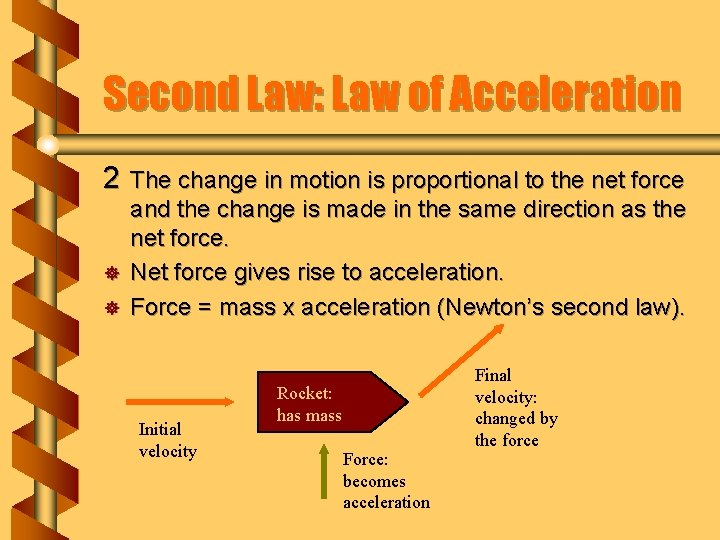Second Law: Law of Acceleration 2 The change in motion is proportional to the net force ] ] and the change is made in the same direction as the net force. Net force gives rise to acceleration. Force = mass x acceleration (Newton’s second law). Initial velocity Rocket: has mass Force: becomes acceleration Final velocity: changed by the force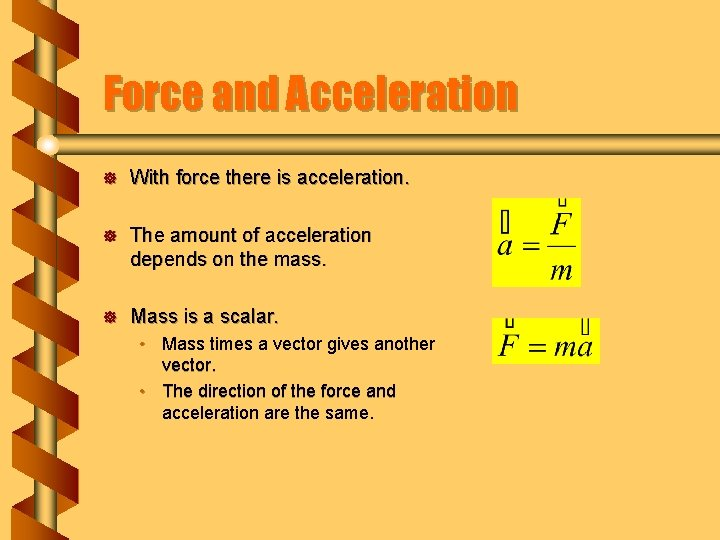Force and Acceleration ] With force there is acceleration. ] The amount of acceleration depends on the mass. ] Mass is a scalar. • Mass times a vector gives another vector. • The direction of the force and acceleration are the same.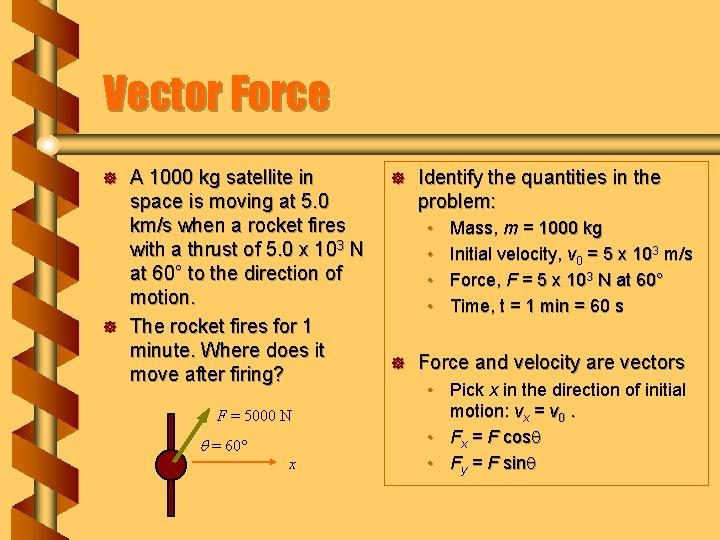Vector Force ] ] A 1000 kg satellite in space is moving at 5. 0 km/s when a rocket fires with a thrust of 5. 0 x 103 N at 60° to the direction of motion. The rocket fires for 1 minute. Where does it move after firing? F = 5000 N q = 60 x ] Identify the quantities in the problem: • • ] Mass, m = 1000 kg Initial velocity, v 0 = 5 x 103 m/s Force, F = 5 x 103 N at 60° Time, t = 1 min = 60 s Force and velocity are vectors • Pick x in the direction of initial motion: vx = v 0. • Fx = F cosq • Fy = F sinq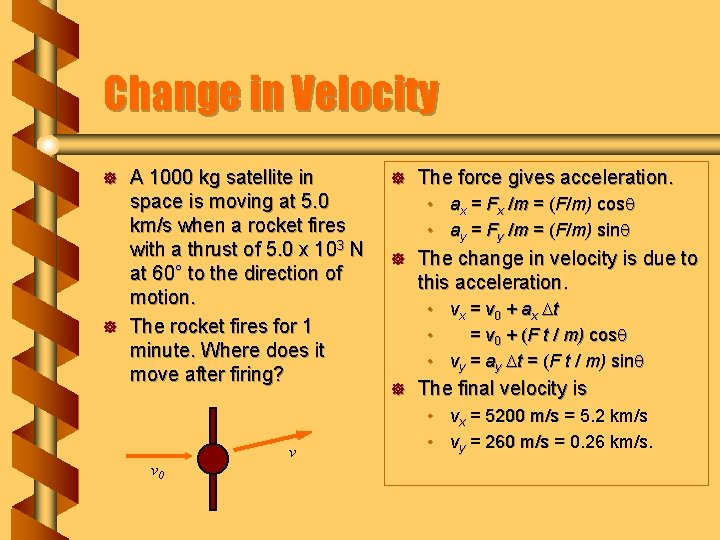Change in Velocity ] ] A 1000 kg satellite in space is moving at 5. 0 km/s when a rocket fires with a thrust of 5. 0 x 103 N at 60° to the direction of motion. The rocket fires for 1 minute. Where does it move after firing? v v 0 ] The force gives acceleration. • ax = Fx /m = (F/m) cosq • ay = Fy /m = (F/m) sinq ] The change in velocity is due to this acceleration. • vx = v 0 + ax Dt • = v 0 + (F t / m) cosq • vy = ay Dt = (F t / m) sinq ] The final velocity is • vx = 5200 m/s = 5. 2 km/s • vy = 260 m/s = 0. 26 km/s.